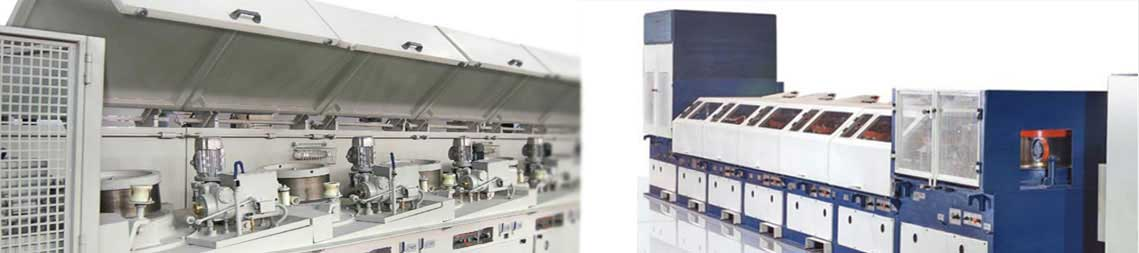# Conversions

The following table shows the conversion factors for commonly used units used in our wire drawing machine industry.

 Conversion Factors For Commonly Used Units Imperial SI Metric From Imperial To SI Metric Multiply by From SI Metric to Imperial Multiply by Linear Measurement Inch Mil Ft. Yd. Mile Mm (millimetre) m (micrometre) m (metre) m km (kilometre) 25.4 25.4 0.304 8 0.914 4 1.609 34 0.039 37 0.039 37 3.280 84 1.093 61 0.621 37 Area Sq. inch Sq. inch Sq. ft. Sq. yd. Mm2 Cm2 M2 M2 645.16 6.451 6 0.092 903 0.836 13 0.01 55 0.155 00 10.763 91 1.195 99 Volume And Liquid Capacity Cu. Inch Cu.ft. Cu.yd. Fluid oz. (lmp.) gallon (lmp) Gallon (US) Cm3 M3 M3 Ml (millilitre) L (litre) L 16.387 06 0.028 37 0.764 55 28.413 06 4.546 09 3.785 41 0.061 024 35.314 67 1.307 95 0.035 195 0.219 97 0.264 17 Weight (mass)… Oz Ib. Ton (Short) G (gram) Kg (kilogram) T (tonne) 28.349 52 0.453 59 0.907 18 0.035 274 2.204 62 1.102 31 Weight per unit length Ib./ft. Kg/m 1.488 16 0.671 97 Coating weight Oz.sq. ft. G/m2 305. 152 0.003 277 Force Pound-force Kip N (newton) Kn (kilonewton) 4.448 22 4.448 22 0.224 81 0.224 81 Pressure And stress*** Psi Ksi Mpa (megapascal) 0.006 8948 6.894 76 145.039 0.145 04 Pressure and stress*** Psi Ksi Kgf/mm2 Kgf/mm2 0.000 7031 0.703 1 1422.347 1.422 347 Torque and bending Ib.in. n.m. (Newton metre) 0.112 99 8.850 75 Moment Ib. ft. n.m. 1.355 82 0.737 56 Energy Ft. lb. J (Joule) 1.355 82 0.737 56 Temperature 0F 0C 5/9 (0F-32) 9/50C+32

Note : Underlined values are exact.

• The SI metric system is based on the international system of units.
• In some circumstances the term "weight" is used as meaning force and, therefore, it would have the units of force i.e. N(newtons). It is a more common practice to associate "Weight" with mass when it would have units of mass. I.e. kg (kilograms).
• Properly, stress is expressed in pascals (PA) or N/m2. However, M pascals or n/mm2 is commonly used.
• It is convert kgf/ mm2 to MPA (N/ mm2), multiply by 9.80665.